#1

## SICP lec6a : 流 I part3 (lazy seq 解釋)

### 找出 1,000 ~ 1,000,000 中的第二個質數

1. map 所有 1,000 ~ 1,000,000 的數字
2. filter 其中的質數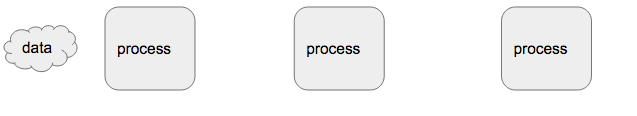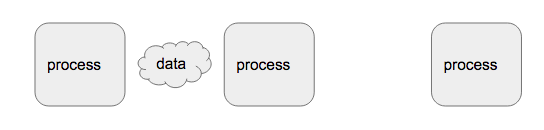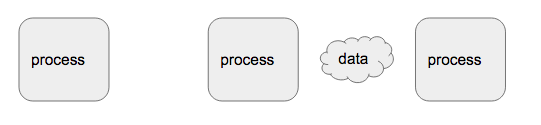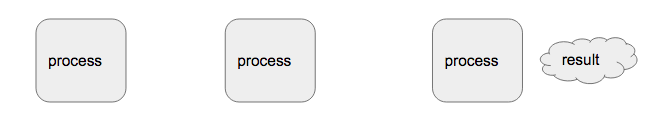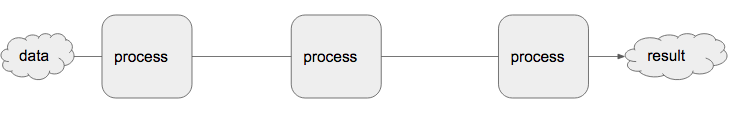``````(defn con-stream [x y]
(cons x (delay y)))
(defn tail [s] (force (cdr s)))
``````
• `delay` 所做的有兩件事 1. 產生function的表達式 2.一個執行許可，當接收到執行許可時再去執行。
• `force` 就是 `delay` 的執行許可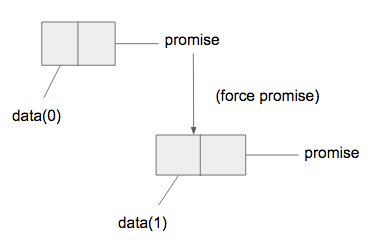#### 回到最一開始的問題，來檢視一下 夢幻流 的執行過程

`(head (tail (filter prime? (map 每一個數 1000 1000000)))))`

`(map 每一個數 1000 1000000) -> (cons 1000 (delay (map 每一個數 1001 1000000)))`

• map第一個數 1000 出來計算
• 其他的先不計算
``````(filter prime? (map 每一個數 1000 1000000))
-> (filter prime? 1000)
-> (filter prime?(tail (cons 1000 (delay (map 每一個數 1001 1000000)))))
``````
• filter prime? 計算第一個數 1000
• 執行tail，force (map 每一個數 1001 1000000)
• 遞迴的對 tail 做 filter prime?
• 產生第一個質數後停止，但要求是第二個因此繼續啟動

``````(defn delay [exp] (fn [] (exp)))
(defn force [fn] (fn))
``````

#### delay 神奇的地方在於將 "預期事件的執行順序" 與 "實際電腦的執行順序" 解耦開來，以至於可以用 "綜觀全局不考慮每個時間狀態" 的表示方式，做出 "最有效率，不佔無效空間" 的執行。

``````(defn delay [exp] (memo-proc (fn [] (exp))))
``````

`memo-proc`是一個function，記錄前面所有產生過的數據，讓每一次force tail時只做一次的計算。